# What Is Trigonometry? Definition and History

Melanie has a BS in physical science and is in grad school for analytics and modeling. She also runs a YouTube channel: The Curious Coder.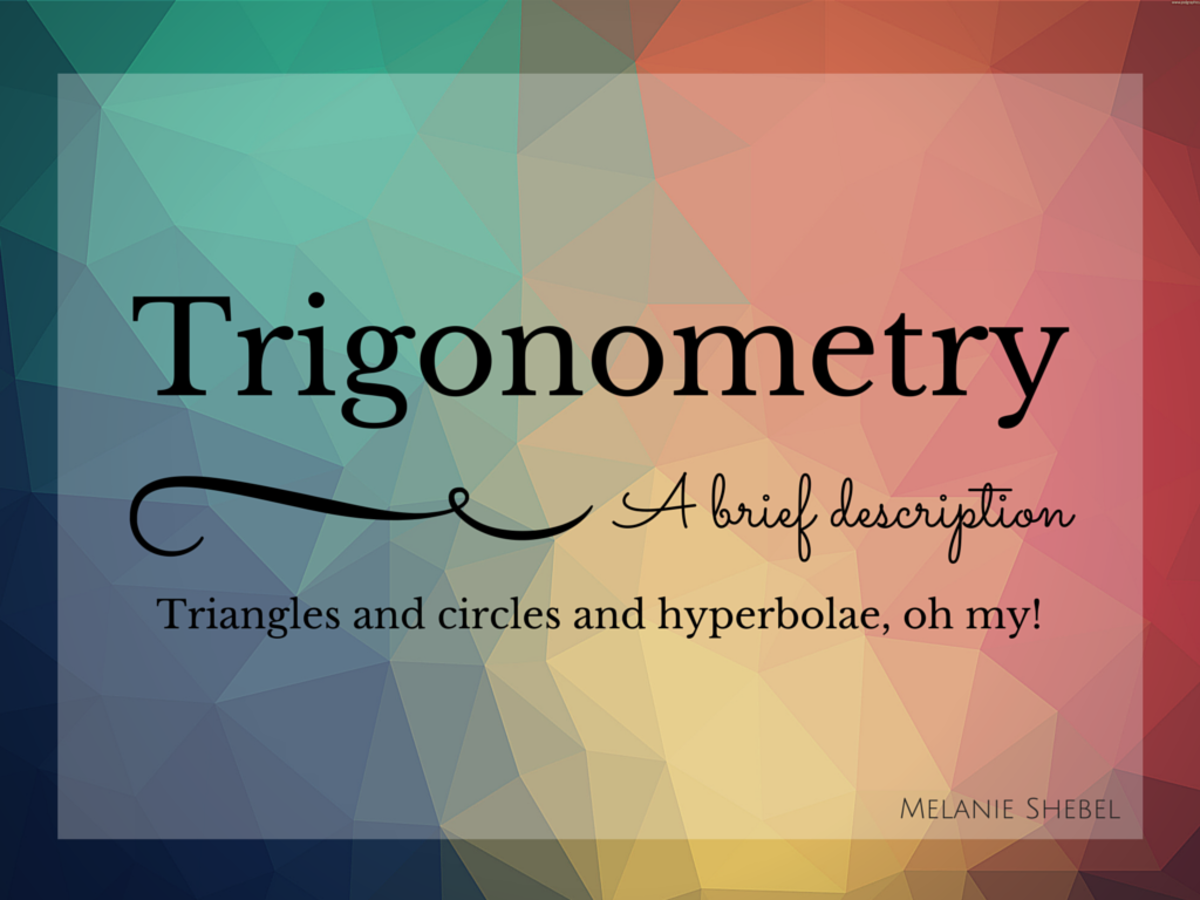Trigonometry, a brief description. Triangles and circles and hyberbolae, oh my!

What Is Trigonometry?

Trigonometry is the branch of mathematics devoted explicitly to the relationship between the sides and angles of triangles. Its name even sounds triangle-y and for a good reason: the word trigonometry is derived from two Greek words - 'trigonon' which means 'triangle' and 'metron' meaning 'measure.' Put together, the words mean "triangle measuring."

## It's More Than Just Triangles

Trigonometry is more than just measuring triangles. It's also circle measuring, hyperbola measuring, and ellipse measuring—things that are decidedly very non-triangular. This can be achieved by the usage of the ratios between the sides and angles of a triangle (which will be discussed later) and the manipulation of variables.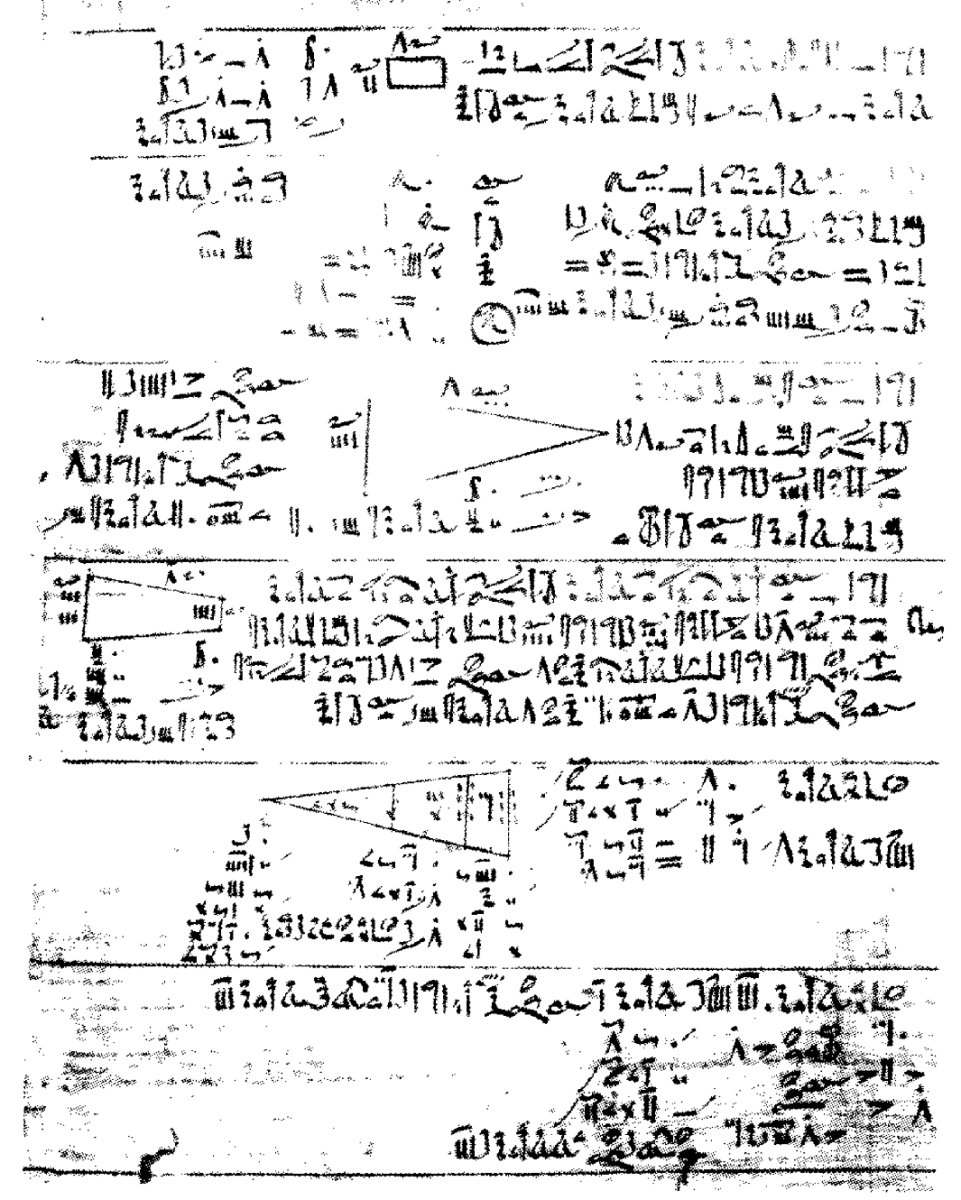A part of the Rhind Mathematical Papyrus showing early trigonometrypublic domain

## The Early Roots of Trigonometry

Defining the very beginning of a concept is difficult. Because mathematics is so abstract, we can't just say a cave painting of a triangle is trigonometry. What did the painter mean by the triangle? Did he just like triangles? Was he enthralled with how the length of one side, another side, and the angle they made dictated the length and angles of the other sides?

Furthermore, paperwork back in the day was notoriously poorly filed and sometimes burned. Also, duplicates were often not made (they didn't have electricity to power copy machines.) In short, stuff got lost.

The earliest known "strong" example of trigonometry is found on the Rhind Mathematical Papyrus which dates to around 1650 BC. The second book of the papyrus shows how to find the volume of cylindrical and rectangular granaries and how to find the area of a circle (which at that time was approximating using an octagon.) Also on the papyrus are calculations for pyramids including a sophisticated approach that uses a beat-around-the-bush method for finding the value of the cotangent of the angle to a pyramid's base and its face.

In the late 6th century BC, the Greek mathematician Pythagoras gave us:

a2 + b2 = c2

The stands as one of the most commonly used relations in trigonometry and is a special case for the Law of Cosines:

c2 = a2 + b2 - 2ab cos(θ)

However, the systematic study of trigonometry dates to the middle ages in Hellenistic India where it began to spread across the Greek empire and bled into Latin territories during the Renaissance. With the Renaissance came an enormous growth of mathematics.

However, it wasn't until the 17th and 18th centuries that we saw the development of modern trigonometry with the likes of Sir Isaac Newton and Leonhard Euler (one of the most significant mathematicians the world will ever know). It is Euler's formula that establishes the fundamental relationships between the trigonometric functions.

## The Trigonometric Functions

In a right triangle, six functions can be used to relate the lengths of its sides with an angle (θ.)

Scroll to Continue

## Read More From Owlcation

The three ratios sine, cosine, and tangent are reciprocals of the ratios cosecant, secant, and cotangent respectively, as shown: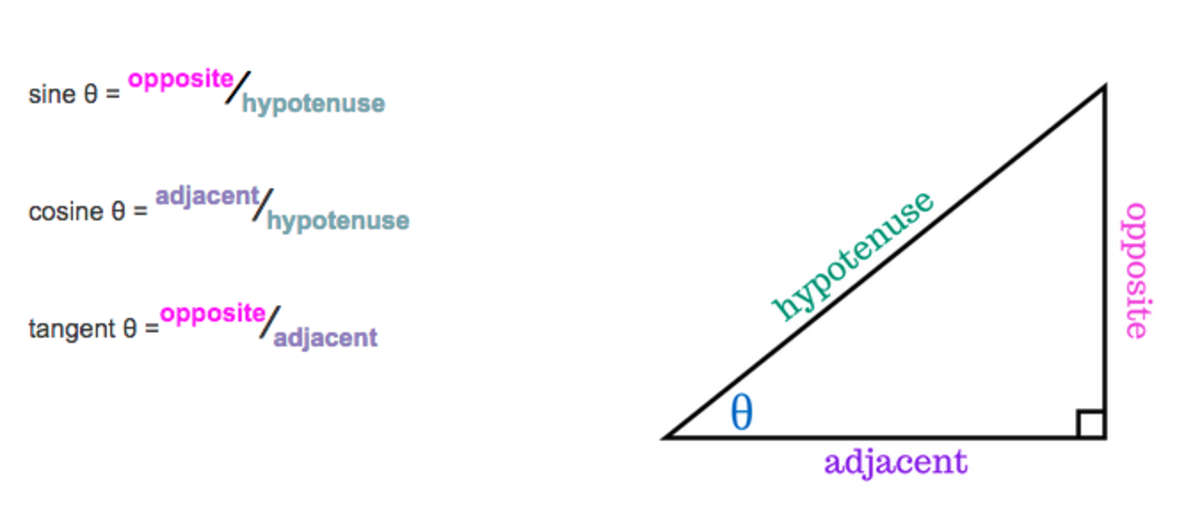The three ratios sine, cosine, and tangent are reciprocals of the ratios cosecant, secant, and cotangent respectively, as shown.Melanie Shebel

If given the length of any two sides, the usage the Pythagorean Theorem not only allows one to find the length of the missing side of the triangle but the values for all six trigonometric functions.

While the usage of the trigonometric functions may seem limited (one might only need to find the unknown length of a triangle in a small number of applications), these tiny pieces of information can be extended much further. For example, right triangle trigonometry can be used in navigation and physics.

For example, sine and cosine can be used to resolve polar coordinates to the Cartesian plane, where x = r cos θ and y = r sin θ.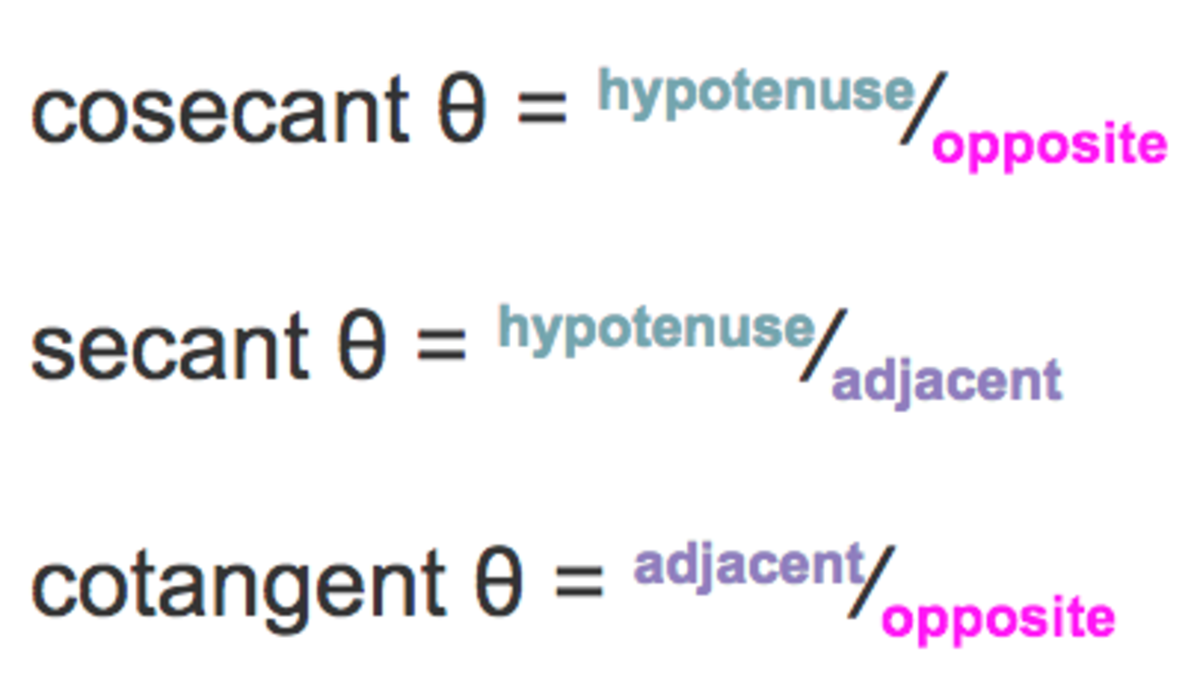The three ratios sine, cosine, and tangent are reciprocals of the ratios cosecant, secant, and cotangent respectively, as shown.Melanie ShebelUsing a right triangle to define a circle.Pbroks13, cc-by-sa, via Wikimedia Commons

## Geometric Curves: Conics in Trig

As mentioned above, trigonometry is powerful enough to make measurements of things that are not triangles. Conics such as hyperbolae and ellipses are examples of how awesomely sneaky trigonometry can be—a triangle (and all its formulae) can be hidden inside an oval!

Let's start with a circle. One of the first things one learns in trigonometry is that the radii and arcs of a circle can be found using a right triangle. This is because the hypotenuse of a right triangle is also the slope of the line connecting the center of the circle with a point on the circle (as shown below). This same point can also be found using the trigonometric functions.

Working with triangles to find information about a circle is easy enough, but what happens with ellipses? They’re just flattened circles, but the distance from the center to the edge is not uniform as it is in a circle.

It could be argued that an ellipse is better defined by its foci than its center (while noting that the center is still useful in calculating the equation for the ellipse). The distance from one focus (F1) to any point (P) added to the distance from the other focus (F2) to point P does not differ as one travels around the ellipse. An ellipse is related using b2 = a2 – c2 where c is the distance from the center to either focus (either positive or negative), a is the distance from the center to the vertex (major axis), and b is the distance from the center to the minor-axis.

## Equations for Ellipses

The equation for an ellipse with center (h,k) where the x-axis is the major axis (as in the ellipse shown below) is: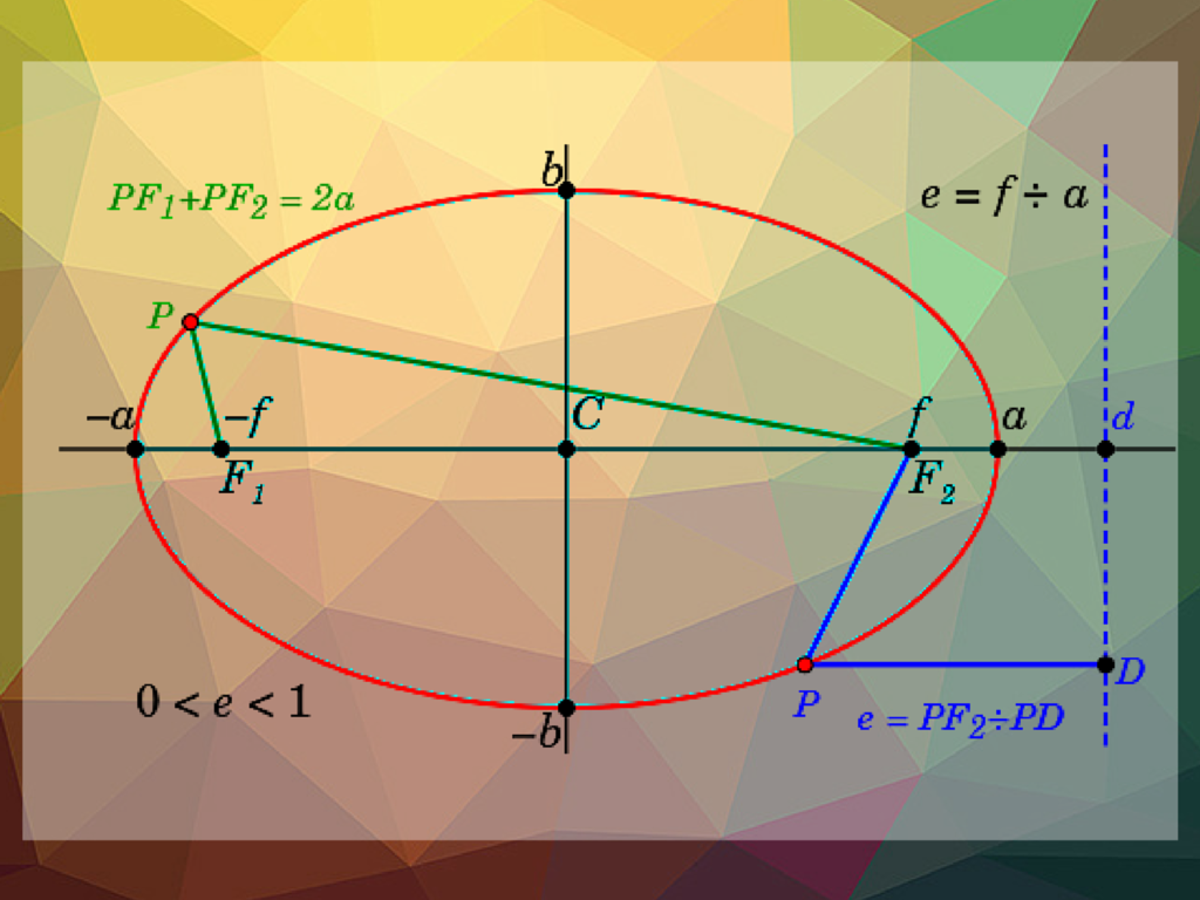An ellipse where the x-axis is the major axis. Vertices at (h,a) and (h,-a).Melanie Shebel

However, the equation for an ellipse where the major axis is the y-axis is related by:

## Equations for Hyperbolae

A hyperbola looks very different from an ellipse. In fact, nearly oppositely so . . . it’s a hyperbola split in half with the halves facing in opposite directions. However, in terms of finding the equations of hyberbolae versus any other “shape,” the two are closely related.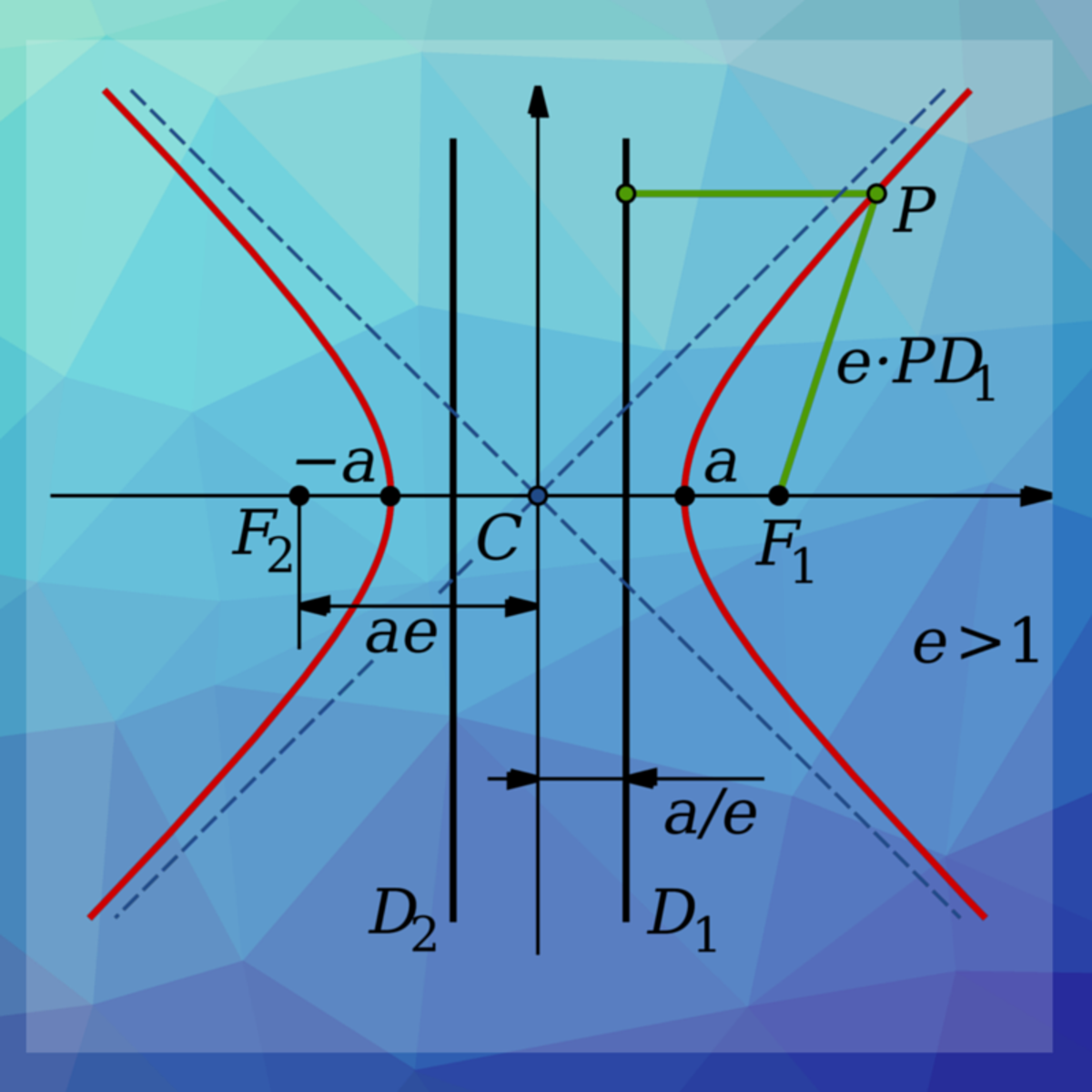A hyperbola transversed across the x-axisMelanie Shebel

Like an ellipse, the center of a hyperbola is referenced by (h,k.) However, a hyperbola only has one vertex (noted by the distance a from the center in either the x or y-direction depending on the transverse axis.)

Also unlike an ellipse, the foci of a hyperbola (noted by distance c from the center) are further from the center than the vertex. The Pythagorean Theorem rears its head here too, where c2 = b2 + a2 using the equations to the right.

As you can see, trigonometry can bring one further than just finding the missing length of a triangle (or a missing angle.) It's used for more than just measuring the height of a tree by the shadow it casts or finding the distance between two buildings given some unusual scenario. Trigonometry can be applied further to define and describe circles and circle-like shapes.

Hyperbolae and ellipses serve as great examples of how trigonometry can quickly deviate from just stating the Pythagorean Theorem and the few relationships between the lengths of the sides of a simple triangle (the trig functions.)

The toolset of equations in trigonometry is small, however, with a bit of creativity and manipulation, these equations can be used to obtain an accurate description of a wide variety of shapes such as ellipses and hyperbolae.

© 2017 Melanie Palen